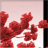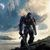Announcements
The way to achieve your own success is the willingness to help somebody else. Go for it!
cancel
Showing results for
Did you mean:Specialist

## Top n in Qliksense straight table

Hi All,

I am facing issue while showing top 50 records in qliksense straight table.

I have applied limit top 50 on dimension it didn't work.

I have written expression using Rank function still it didn't work.

I have 5 dimensions and 7 expressions in table.

I have tried below expressions,

=if(rank(\$(exp_Sales_Delta)) <= 50,\$(exp_SalesGbp))

=if(rank(\$(exp_SalesGbp)) <= 50,\$(exp_SalesGbp))

even on one of the expression,

=if(rank(\$(exp_SalesGbp)) <= 50, Aggr(\$(exp_SalesGbp), [Issuer Name]))

Labels (2)

• ### Visualization

12 RepliesMaster

Possibly as a calculated dimension while suppressing null values.

=Aggr(If(Rank(\$(exp_SalesGbp))<=50, [Issuer Name]), [Issuer Name])Specialist
Author

do we need to do this for all the dimensions? as, with only this one it's not working.Master

What is this "exp_SalesGbp" variable made up of? Post some sample.Creator

here iam giving you an example expression and i hope it works for you.

aggr(if(rank(sum([Sales]))<=5, City), City)

Please like and accept the solution if u you find an answerSpecialist
Author

this is like, variable for current cob sales

Replace(Replace('sum({<[COB Date] = {^~(v_CurrentCOB)^}>} [Sales Gbp])','~','\$'),'^',Chr(39))Specialist
Author

this is like, variable for current cob sales

Replace(Replace('sum({<[COB Date] = {^~(v_CurrentCOB)^}>} [Sales Gbp])','~','\$'),'^',Chr(39))Specialist
Author

this one also I have tried but, seems not workingMaster

Hello, you don't have to apply to every dimension that is used.

But first of all replace \$(exp_SalesGbp)) with \$(=\$(exp_SalesGbp)))MVP

Try this

=Aggr(If(Rank(\$(exp_SalesGbp)) <= 50, \$(exp_SalesGbp)), [YourDimension])Tags
Community Browser